# What is Birefringence and Beat Length?

### >> What is Birefringence in Optical Fiber?

The analysis of the guiding properties of single mode fibers leads to the propagation of one transversal mode called LP01, or, more precisely, two orthogonal degenerate modes LP01x and LP01y.

The term degenerate means that both modes are characterized by the same propagation constant, i.e. the same effective refractive index (nx = ny).

However, this degeneracy is theoretical: nx = ny holds only for an optical fiber with a perfect circular symmetry which can never be obtained in practice. The core is indeed always slightly elliptical rather than circular.

Moreover, the dopant concentration in the core section is never perfectly uniform and the fiber material can be subjected to stress or bending.

The effect of the non-circular symmetry is to remove the degeneracy of modes LP01x and LP01y. Consequently, their effective refractive indices are different (nx  ny) and the fiber exhibits birefringence. This leads to two different group velocities of the modes and we can already feel that the birefringence will lead to a dispersive phenomenon.

The birefringence, as a parameter, is defined as the local absolute value of the difference between the propagation constants of both modes: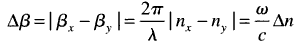where c is the speed of the light in vacuum and Δn is the refractive index difference, also called the degree of birefringence. In standard fibers, the degree of birefringence is about 10-7.

If we assume that the fiber is characterized by a uniform birefringence along its length L (Δβ is constant), the absolute phase delay between the two polarization modes can be written as: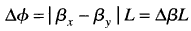Since the light components of the fast and slow eigenmodes propagate with different velocities, an optical pulse launched in the fiber will be broadened during the propagation. The difference in group velocities leads to a differential group delay (DGD) Δτ, illustrated in the following figure for a linear birefringent element for which the two eigenmodes are linear.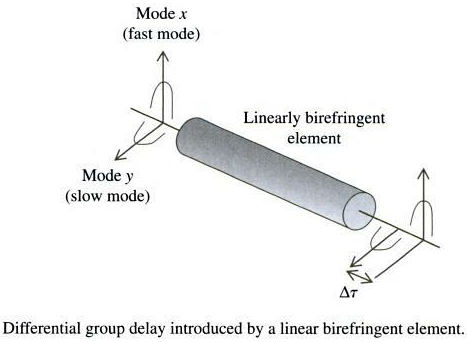The expression for Δτ is obtained by taking the derivative of the previous equation with respect to ω: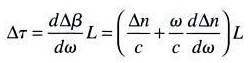### >> What is Optical Fiber Beat Length?

The birefringence also modifies the state of polarization while it propagates in the fiber. Take a look at the following figure.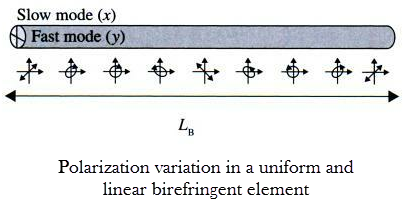If we launch in the fiber section a linear 45° SOP (State of Polarization), it can be decomposed into two contributions: one aligned with the slow mode (x axis) and the other aligned with the fast mode (y axis).

The output SOP will depend on the phase difference between the phases of the x and y components. Since the birefringent element modifies the phase difference between them, it clearly appears that the presence of birefringence modifies the polarization state.

The above figure shows how the SOP varies. The right-handed circular state is obtained when the phase delay generated by the fiber is π/2. The 135° linear state is obtained when Δφ = π. When the phase delay reaches 2π, the SOP is identical to that of the input. This condition is obtained after a propagation length, called the Beat Length (denoted by LB) such that: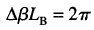This yields: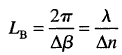Typical values of LB for standard single mode fibers are between 1 meter and 50 meters. If the polarization state of the input light corresponds to one of the fiber eigenmodes (and in the absence of mode coupling), it will maintain its polarization state as it propagates down the fiber.

This property is used for maintaining polarization in an optical fiber system by using a PMF fiber (Polarization-Maintaining Fiber), where a high value of Δn minimizes coupling between the two modes.